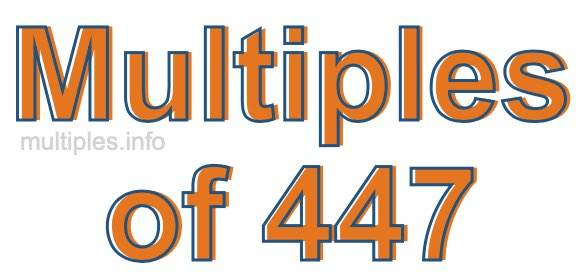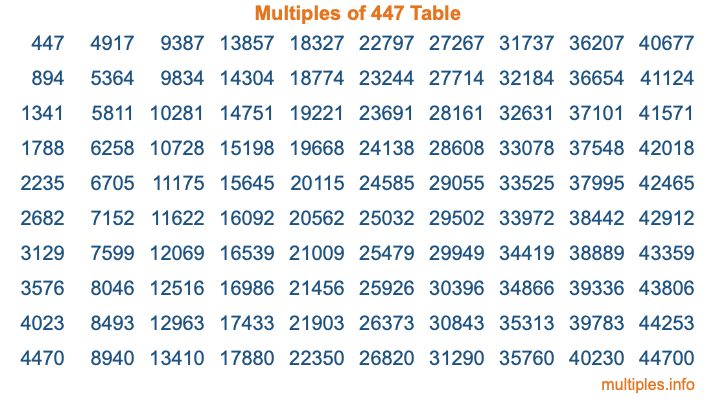Multiples of 447Welcome to the Multiples of 447 page. Here we will first teach you everything you will ever need to know about the multiples of 447, and then give you a study guide summary of everything we taught you to make sure you remember it all. Use this page to look up facts and learn information about the multiples of 447. This page will make you a multiples of four hundred forty-seven expert!

Definition of Multiples of 447
Multiples of 447 are all the numbers that when divided by 447 equal an integer. Each of the multiples of 447 are called a multiple. A multiple of 447 is created by multiplying 447 by an integer.

Therefore, to create a list of multiples of 447, you start with 1 multiplied by 447, then 2 multiplied by 447, then 3 multiplied by 447, and so on for as long as you want. Thus, the list of the first five multiples of 447 is 447, 894, 1341, 1788, and 2235. To see a larger list of multiples of 447, see the printable image of Multiples of 447 further down on this page. We also have a category where you can choose any nth multiple of 447.

Multiples of 447 Checker
The Multiples of 447 Checker below checks to see if any number of your choice is a multiple of 447. In other words, it checks to see if there is any number (integer) that when multiplied by 447 will equal your number. To do that, we divide your number by 447. If the the quotient is an integer, then your number is a multiple of 447.

Is  a multiple of 447?

Least Common Multiple of 447 and ...
A Least Common Multiple (LCM) is the lowest multiple that two or more numbers have in common. This is also called the smallest common multiple or lowest common multiple and is useful to know when you are adding our subtracting fractions. Enter one or more numbers below (447 is already entered) to find the LCM.

Check out our LCM Calculator if you need more details about the Least Common Multiple or if you need the LCM for different numbers for adding and subtraction fractions.

nth Multiple of 447
As we stated above, 447 is the first multiple of 447, 894 is the second multiple of 447, 1341 is the third multiple of 447, and so on. Enter a number below to find the nth multiple of 447.

th multiple of 447

Multiples of 447 vs Factors of 447
447 is a multiple of 447 and a factor of 447, but that is where the similarities end. All postive multiples of 447 are 447 or greater than 447. All positive factors of 447 are 447 or less than 447.

Below is the beginning list of multiples of 447 and the factors of 447 so you can compare:

Multiples of 447: 447, 894, 1341, 1788, 2235, etc.

Factors of 447: 1, 3, 149, 447

As you can see, the multiples of 447 are all the numbers that you can divide by 447 to get a whole number. The factors of 447, on the other hand, are all the whole numbers that you can multiply by another whole number to get 447.

It's also interesting to note that if a number (x) is a factor of 447, then 447 will also be a multiple of that number (x).

Multiples of 447 vs Divisors of 447
The divisors of 447 are all the integers that 447 can be divided by evenly. Below is a list of the divisors of 447.

Divisors of 447: 1, 3, 149, 447

The interesting thing to note here is that if you take any multiple of 447 and divide it by a divisor of 447, you will see that the quotient is an integer.

Multiples of 447 Table
Below is an image of the first 100 multiples of 447 in a table. The table is in chronological order, column by column. The first column has the first ten multiples of 447, the second column has the next ten multiples of 447, and so on.The Multiples of 447 Table is also referred to as the 447 Times Table or Times Table of 447. You are welcome to print out our table for your studies.

Negative Multiples of 447
Although not often discussed or needed in math, it is worth mentioning that you can make a list of negative multiples of 447 by multiplying 447 by -1, then by -2, then by -3, and so on, to get the following list of negative multiples of 447:

-447, -894, -1341, -1788, -2235, etc.

Multiples of 447 Summary
Below is a summary of important Multiples of 447 facts that we have discussed on this page. To retain the knowledge on this page, we recommend that you read through the summary and explain to yourself or a study partner why they hold true.

There are an infinite number of multiples of 447.

A multiple of 447 divided by 447 will equal a whole number.

447 divided by a factor of 447 equals a divisor of 447.

The nth multiple of 447 is n times 447.

The largest factor of 447 is equal to the first positive multiple of 447.

447 is a multiple of every factor of 447.

447 is a multiple of 447.

A multiple of 447 divided by a divisor of 447 equals an integer.

447 divided by a divisor of 447 equals a factor of 447.

Any integer times 447 will equal a multiple of 447.

Multiples of a Number
Here you can get the multiples of another number, all with the same attention to detail as we did for multiples of 447 on this page.

Multiples of
Multiples of 448
Did you find our page about multiples of four hundred forty-seven educational? Do you want more knowledge? Check out the multiples of the next number on our list!